• 线性卷积循环卷积 在音频信号处理中，卷积是很常见的信号处理方式，例如fir滤波器，卷积的计算公式也非常简单，对于系统h输入信号x，卷积的计算公式如下：
线性卷积与循环卷积
在音频信号处理中，卷积是很常见的信号处理方式，例如fir滤波器，卷积的计算公式也非常简单，对于系统h和输入信号x，卷积的计算公式如下：
$y(t)=\sum_{m=0}^{N-1}x(t-m)h(m)$
$y(t) = [\sum_{m=0}^{N-1}x(t-m)h(m)]R_N(t)$
而一个N点循环卷积相当于将x和h做周期为N的延拓后进行卷积，然后再取其主值区间，得到的结果长度为N
我们定义$x=[1,2,3,4]$和$h=[5,6,7,8]$，下面用一张示意图来表示线性卷积和圆周卷积的计算方式：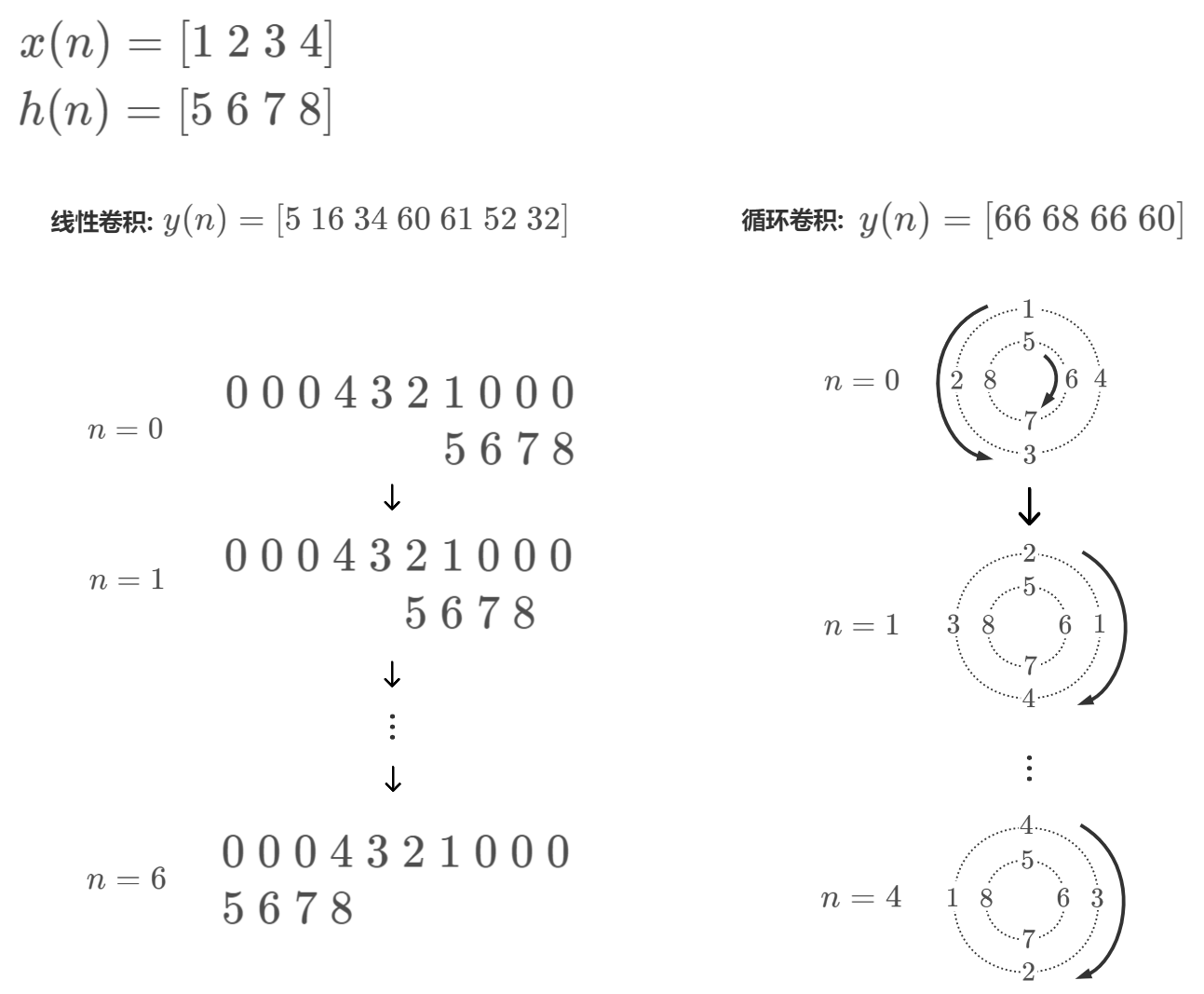总结下来就是：
线性卷积：将x补零，反褶，然后滑动，与对应位置的h相乘求和
循环卷积：将x和h补零至N点，x逆时针平均分布在大圆上，h顺时针分布在小圆上，然后顺时针旋转大圆，与对应位置的h相乘求和
我们可以将卷积中每一步的滑动变换为矩阵乘法并行的进行卷积运算，对于循环卷积，将其变换为矩阵后是一个循环矩阵，我们利用python代码来求线性卷积和循环卷积：
import numpy as np
from scipy.linalg import toeplitz

def circular_convolve(x,h,N):
r = np.roll(c[::-1],1)
x_c = toeplitz(c,r)
return np.dot(x_c,h_c)

def convolve(x,h):
L = len(x)
M = len(h)
N = L+M-1
index = np.arange(M)[::-1][None, :] + np.arange(N)[:, None]
return np.dot(x_p[index],h)


x = [5,6,7,8]
h = [1,2,3,4]
L=4

print('线性卷积结果为:')
print(convolve(x,h))
print('循环卷积结果为:')
print(circular_convolve(x,h,L))

输出为：
线性卷积结果为:
[ 5 16 34 60 61 52 32]
循环卷积结果为:
[66 68 66 60]

卷积与FFT
虽然卷积的计算方法很简单，但是当x和h都很长的时候，卷积计算是非常耗费时间的。直接卷积运算的复杂度为$\mathcal{O}[N^2]$，因此有必要找到比直接计算卷积更快的办法。
相信大家都知道时域的卷积等于频域的乘积这个定理，因此要计算时域的卷积，可以将时域信号转换为频域信号，进行乘积运算之后再将结果转换为时域信号，实现快速卷积。
在我之前的文章使用python实现基-2FFT、基-4FFT快速傅里叶变换算法中分析到，经过优化的FFT其运算的复杂度为$\mathcal{O}[NlogN]$，显然通过FFT计算卷积要比直接计算快速得多。
不过由于FFT运算假设其所计算的信号为周期信号，因此通过FFT计算出的结果实际上是两个信号的循环卷积，而不是线性卷积。
使用numpy的fft来计算卷积：
import numpy as np

x = [5,6,7,8]
h = [1,2,3,4]

X = np.fft.rfft(x)
H = np.fft.rfft(h)
y = np.fft.irfft(X*H)

print('fft计算卷积结果为:')
print(y)

输出为：
fft计算卷积结果为:
[66. 68. 66. 60.]

可以看到，将x和h变换到频域相乘，然后变换回时域的结果与循环卷积的结果一致。
如果需要使用FFT计算线性卷积，就需要对信号进行补零扩展，使得其长度长于线性卷积结果的长度。
对x和h进行补零，然后再进行fft：
import numpy as np

x = [5,6,7,8]
h = [1,2,3,4]

X = np.fft.rfft(x,n=7)
H = np.fft.rfft(h,n=7)
y = np.fft.irfft(X*H,n=7)

print('fft计算卷积结果为:')
print(y)

输出为：
fft计算卷积结果为:
[ 5. 16. 34. 60. 61. 52. 32.]

可以看到，结果与线性卷积结果一致


展开全文python fft 数字信号处理
• 在DFT的应用中，利用DFT的循环卷积定理进行线性卷积的快速运算这一应用是极其重要的，理解这一重要应用，才能更加清晰理解DFT的快速运算---FFT的作用。本文将从原理入手，给出实际例子，为你细致讲解用DFT实现线性...
引言：在前面的文章中，我们理解了DTFT与DFT的关系，接下来就是要研究DFT的应用。在DFT的应用中，利用DFT的循环卷积定理进行线性卷积的快速运算这一应用是极其重要的，理解这一重要应用，才能更加清晰理解DFT的快速运算---FFT的作用。本文将从原理入手，给出实际例子，为你细致讲解用DFT实现线性卷积快速运算的过程！首先交代背景，我们知道，在实际的应用中，计算一个离散时间系统的输出为：输入离散时间序列和系统单位脉冲响应的线性卷积。然而，线性卷积的计算量是很大的，这牵制了计算机的运算速度，因此我们希望运用循环卷积定理，借助DFT的快速算法FFT，从循环卷积和线性卷积的关系入手，解决这一问题，快速得到系统的输出。一.循环卷积定理循环卷积定理指的是：两个有限长序列循环卷积的结果得DFT，等于这两个序列单独做DFT后的乘积，具体公式为下图：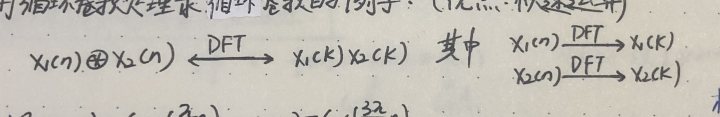之所以引入这一定理，是因为DFT存在一种快速运算---FFT（后面文章会讲到），FFT可以把计算时间大大缩短。因此只要计算出两个序列的DFT，将他们相乘，再把结果作IDFT，就可以得到两个有限长序列的循环卷积。注意！我们的目标是求两个序列的线性卷积，因此接下来的任务是找到循环卷积的关系！二.循环卷积和线性卷积的关系线性卷积和循环卷积的关系要由周期卷积做过度，周期卷积为，假设长度为N，长度为M，则选取max(N,M)，以L为周期将两序列线性卷积的结果周期延拓，然后重叠部分相加，留下对应的L个点，所得即为周期卷积结果。可见当N+M-1(线性卷积长度）时，周期卷积结果等于线性卷积结果。而两个序列循环卷积的结果长度为周期卷积后取主值（取值的点数为循环卷积的点数）。因此，当给两个序列末尾补零，使得两个序列长度都为N+M-1，此时，做循环卷积的结果就等于线性卷积的结果。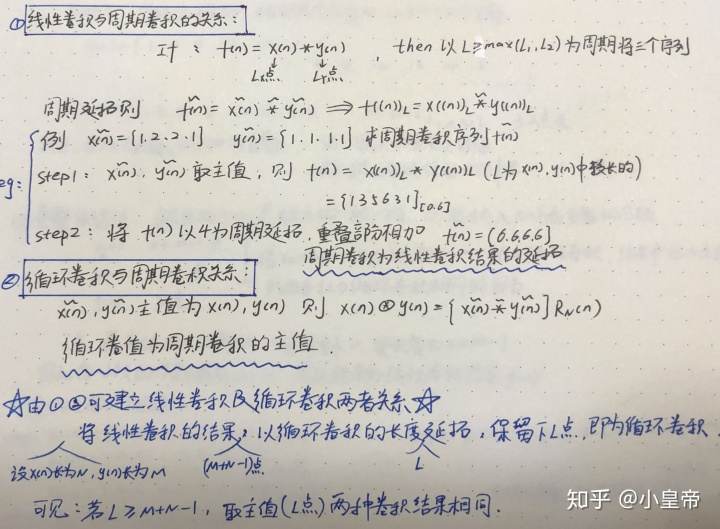注意笔记中波浪线的部分，可借助具体例子理解三.有限长序列与有限长序列的线性卷积计算实际系统中，我们的系统的系统单位冲脉冲响应是确定的，假设它是有限长的，当输入也为有限长时，可用如下方法计算：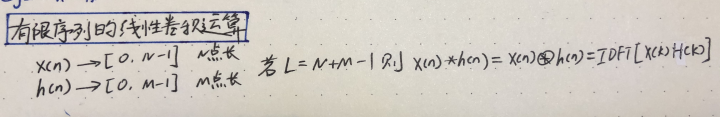也就是，等待输入都输入完成，对两序列补零，利用循环卷积和线性卷积关系做运算，最后做IDFT得到结果四.无限长与有限长序列的线性卷积计算如果输入是一个很长很长的序列，我们不可能等所有的点都输入进来之后，再对序列补零计算，这样等待输入的时间是很长的，对于这样的情况，我们有重叠相加法和重叠保留法，这两种方法只是对输入的切割方式不同，总体思想都是分割输入，转换为一段一段的有限长序列来计算，带来的结果是一样的，下面笔记中给出的实际例子说明了这一点！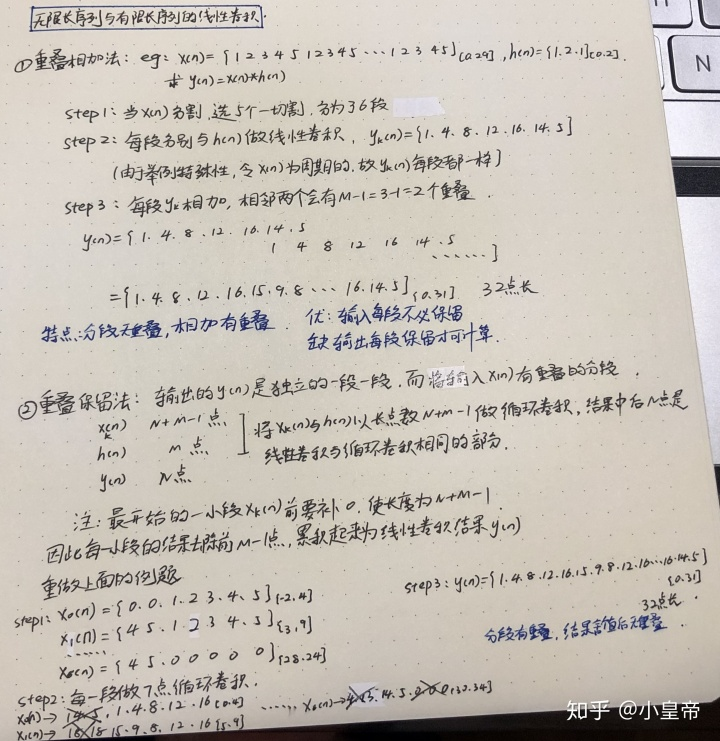可见两种方法得到的结果是一样的至此，我们已经知道了如何借助循环卷积和线性卷积的关系，通过循环卷积定理，利用FFT快速计算系统的输出了（无论是有限长的输入还是无限长的输入），在后面的文章中，将学习FFT的具体操作方法。
展开全文• 线性卷积就是用h去加权x各个延时版本再求和，其中...因此在OFDM系统中添加CP之后，线性卷积就变化为循环卷积，经过信道就相当于对每个频率进行加权，这样话我们就不需要用那么复杂均衡技术了，只需要估计出H(...
线性卷积就是用h去加权x的各个延时版本再求和，其中的延时指的是线性延时。

循环卷积就是用h去加权x的各个循环移位版本，再求和。

DFT中信道转移函数公式，是对于循环卷积成立的，时域的循环卷积才对应频域的乘积。

CP把线性卷积变成循环卷积。因此在OFDM系统中添加CP之后，线性卷积就变化为循环卷积，经过信道就相当于对每个频率进行加权，这样的话我们就不需要用那么复杂的均衡技术了，只需要估计出H(k)的值，然后逆运算就可以恢复原来的符号了，我们是用了循环前缀这一技术才能达到上面所说的效果。我们把星座映射后的符号X=[X1,X2...,Xn]经过IFFT运算后，得到了时域信号x=[x1,x2...,xn],我们加上CP后变成x'，把它变成‘宏观’的信号经过信道，整体上是一个线性卷积没错！但是在原始时域信号x那个小区间内，却始终保持的是循环卷积(只要CP长度大于信道冲激响应长度)，那么我们就可以利用手段来获取信道特征H(k),每个k就对于每个子载波上的符号Xk，所以一个频率选择性信道就变成了多个平坦的信道了。

Cp在OFDM中的作用主要有两点：1）消除ISI 2）消除ICI

对于第一点，当信道的冲击响应长度小于CP长度时，延时的前一个符号没有机会进入后一个符号的区间来影响。

对于第二点，添加CP相当于与原符号进行改造使线性卷积变为循环卷积，保证了R（k）= S(k) * H(k),这里的k就对应一个载波，我们可以直观的理解吧信道划分N个平坦的信道，每个子载波间没有干扰。这样就降低了均衡的复杂度。
展开全文• 线性卷积（linear convolution）是在时域描述线性系统输入输出之间关系的一种运算。 1. 背景   对于线性时不变离散时间系统来说，若序列x(n)x(n)x(n)是系统输入，h(n)h(n)h(n)是系统在单位脉冲作用下单位...
文章目录一、线性卷积1. 应用背景2. 定义式3. 计算方法3.1 定义式3.2 作图法3.3 列表法二、循环卷积1. 序列的循环移位2. 循环卷积的定义3. 用矩阵计算循环卷积的公式三、线性卷积和循环卷积之间的关系四、用DFT计算循环卷积五、用DFT计算线性卷积六、MATLAB实现1. 利用MATLAB的conv()函数计算线性卷积2. 利用矩阵相乘原理计算循环卷积3. 调用fft()函数计算循环卷积与线性卷积
一、线性卷积
线性卷积（linear convolution）是在时域描述线性系统输入和输出之间关系的一种运算。
1. 应用背景
对于线性时不变离散时间系统来说，若序列$x(n)$是系统的输入，$h(n)$是系统在单位脉冲作用下的单位脉冲响应，由于输入离散时间序列$x(n)$可表示为一系列脉冲的线性组合，根据线性系统的齐次性和可加性，$x(n)$作用于系统所引起的零状态响应$y(n)$就是序列$x(n)$与$h(n)$的卷积和。
2. 定义式

$y(n)=x(n)*h(n)=\sum_{k=-∞}^{∞}x(k)h(n-k)$

上式在运算过程中存在序列的翻转、移位、相乘和相加，所以称为卷积和。
3. 计算方法
3.1 定义式
3.2 作图法

步骤：

（1）将序列$x(n)$、$h(n)$的自变量用$k$代换，然后将序列$h(k)$以纵坐标为轴线反转，成为$h(-k)$；
（2）序列$h(-k)$沿$k$轴正方向平移$n$个单位，成为$h(n-k)$；
（3）求乘积$x(k)h(n-k)$；
（4）按定义式求各乘积之和。
3.3 列表法
由$y(n)=x(n)*h(n)=\sum_{k=-∞}^{∞}x(k)h(n-k)$可见，求和符号内$x(k)$的序号$k$与$h(n-k)$的序号$n-k$之和恰好等于$k$。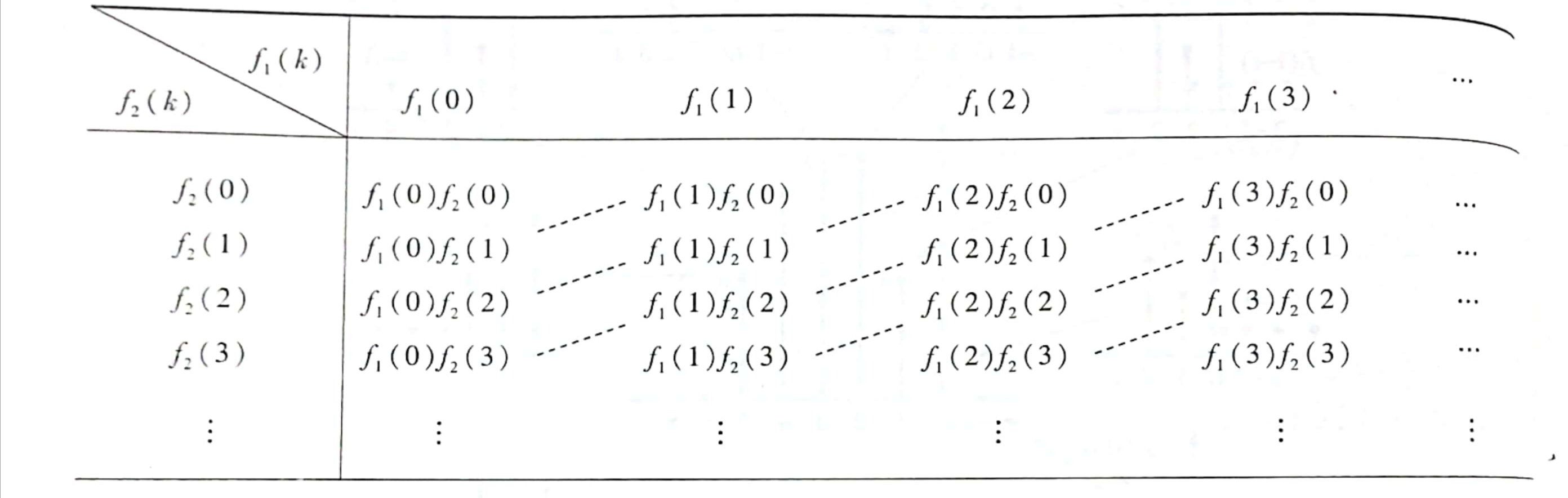沿斜线上各数值之和就是卷积和。

二、循环卷积
1. 序列的循环移位
设$x(n)$为有限长序列，长度为M，M≤N，则$x(n)$的循环移位定义为：
$y(n)=x\big((n+m)\big)_N R_N(n)$
该式表明，将$x(n)$以N为周期进行周期延拓得到$\tilde{x}(n)=x\big((n)\big)_N$，再将$\tilde{x}(n)$左移m得到$\tilde{x}(n+m)=x\big((n+m)\big)_N$，最后取$\tilde{x}(n+m)$的主值序列则得到有限长序列$x(n)$的循环移位序列$y(n)$。$y(n)$是长度为N的有限长序列。
clear all;
close all;
clc;
%%%%%%%%%%%%%%% x(n)及其循环移位过程 %%%%%%%%%%%%%
M=24;
xn=triang(6);
n=-M/3:2*M/3-1;
x=zeros(1,M);
for l=1:6
x(8+l)=xn(l);
end
subplot(4,2,1);
stem(n,x,'.'); axis([-8,16,0,1]); title('(a) x(n)');
b=[xn' 0 0];
y8=[b,b,b];
subplot(4,2,3);
stem(n,y8,'.'); axis([-8,16,0,1]); title('(b) x((n))_8')
y8=[y8 b];
for l=1:M'
ym(l)=y8(l+2);
end
subplot(4,2,5);
stem(n,ym,'.'); axis([-8,16,0,1]); title('(c) x((n+2))_8');
for l=9:16
x(l)=ym(l);
end
subplot(4,2,7);
stem(n,x,'.'); axis([-8,16,0,1]); xlabel('n'); title('(d) x((n+2))_8R_8(n)');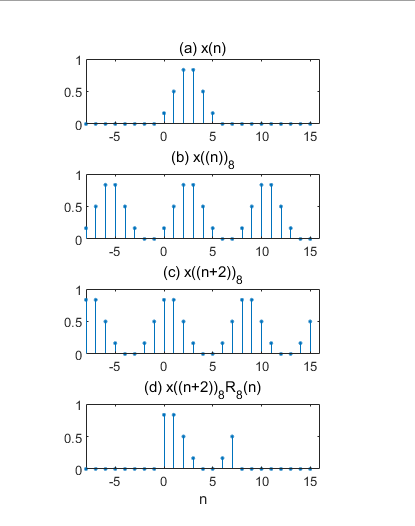2. 循环卷积的定义

设序列$h(n)$和$x(n)$的长度分别为N和M，$h(n)$与$x(n)$的L点循环卷积定义为：
$y_c(n)=\Big[\sum_{m=0}^{L-1}h(m)x\big((n-m)\big)_L\Big]R_L(n)$
式中，L称为循环卷积区间长度，$L≥max[N,M]$，$x\big((n-m)\big)_L$是以L为周期的周期信号，n和m的变化区间均是$[0，L-1]$。
因此直接计算该式比较麻烦，计算机中采用矩阵相乘或FFT的方法计算循环卷积。

3. 用矩阵计算循环卷积的公式
当$n=0,1,2,\cdots,L-1$时，由$x(n)$形成的序列为$\{x(0),x(1),x(2),\cdots,x(L-1)\}$。
令$n=0,m=0,1,2,\cdots,L-1$，$x\big((n-m)\big)_L$形成的序列为：
\begin{aligned} &\{x((0))_L,x((-1))_L,x((-2))_L,\cdots,x((-L+1))_L\}\\ &=\{x(0),x(L-1),x(L-2),\cdots,x(1)\} \end{aligned}
与序列$x(n)$进行对比，相当于将第一个序列值$x(0)$保持不变，将后面的序列$\{x(1),x(2),\cdots,x(L-1)\}$反转180°再放到$x(0)$的后面。这样形成的序列称为$x(n)$的循环倒相序列。
令$n=1,m=0,1,2,\cdots,L-1$，$x\big((n-m)\big)_L$形成的序列为：
\begin{aligned} &\{x((1))_L,x((0))_L,x((-1))_L,\cdots,x((-L+2))_L\}\\ &=\{x(1),x(0),x(L-1),\cdots,x(2)\} \end{aligned}
观察上式等号右端序列，它相当于$x(n)$的循环倒相序列向右循环移一位，即向右移1位，移出区间$[0，L-1]$的序列值再从左边移进。
依次类推，当 n 和 m 均从 0 变化到 L-1 时，得到一个关于$x((n-m))_L$的矩阵：
$\begin{bmatrix} x(0) & x(L-1) & x(L-2)& \cdots& x(1)\\ x(1)& x(0) & x(L-1) & \cdots & x(2)\\ x(2)&x(1)& x(0) & \cdots & x(3)\\ \vdots& \vdots &\vdots &\vdots& \vdots \\ x(L-1)&x(L-2)& x(L-3)& \cdots & x(0)\\ \end{bmatrix}$
上面矩阵称为x(n)的L点“循环卷积矩阵”，其特点是：

第一行是序列$\{x(0),x(1),x(2),\cdots,x(L-1)\}$的循环倒相序列。注意，如果$x(n)$的长度M<L，则需要在$x(n)$末尾补 L-M 个零后，再形成第一行的循环倒相序列；
第一行以后的各行均是前一行向右循环移1位形成的；
矩阵的各主对角线上的序列值均相等。

有了循环卷积矩阵，就可以写出计算循环卷积的矩阵形式：
$\begin{bmatrix} y_c(0)\\ y_c(1)\\ y_c(2)\\ \vdots\\ y_c(L-1)\\ \end{bmatrix} =\begin{bmatrix} x(0) & x(L-1) & x(L-2)& \cdots& x(1)\\ x(1)& x(0) & x(L-1) & \cdots & x(2)\\ x(2)&x(1)& x(0) & \cdots & x(3)\\ \vdots& \vdots &\vdots &\vdots& \vdots \\ x(L-1)&x(L-2)& x(L-3)& \cdots & x(0)\\ \end{bmatrix} \begin{bmatrix} h(0)\\ h(1)\\ h(2)\\ \vdots\\ h(L-1)\\ \end{bmatrix}$
上式中如果$h(n)$的长度N<L，则需要在$h(n)$末尾补上 L-N 个零。
三、线性卷积和循环卷积之间的关系

假设$h(n)$和$x(n)$都是有限长序列，长度分别为N和M。它们的线性卷积和循环卷积分别表示为：
$y_l(n)=h(n)*x(n)=\sum_{m=0}^{N-1}h(m)x(n-m)$
$y_c(n)=\Big[\sum_{m=0}^{L-1}h(m)x\big((n-m)\big)_L\Big]R_L(n)$
其中，$L≥max[N,M]$，$x((n))_L=\sum_{i=-∞}^{∞}x(n+iL)$
所以\begin{aligned} y_c(n)&=\Big[\sum_{m=0}^{N-1}h(m)\sum_{i=-∞}^{∞}x(n-m+iL)\Big]R_L(n)\\ &=\Big[\sum_{i=-∞}^{∞}\sum_{m=0}^{N-1}h(m)x(n+iL-m)\Big]R_L(n) \end{aligned}
与$y_l(n)$式对照可以看出
$\sum_{m=0}^{N-1}h(m)x(n+iL-m)=y_l(n+iL)$
即$y_c(n)=\Big[\sum_{i=-∞}^{∞}y_l(n+iL)\Big]R_L(n)$
也就是说，$y_c(n)$等于$y_l(n)$以L为周期的周期延拓序列的主值序列。

线性卷积与循环卷积相等的条件

$y_l(n)$的长度为N+M-1,因此只有当循环卷积长度L≥N+M-1时，$y_l(n)$以L为周期进行周期延拓时才无时域混叠现象，此时取其主值序列显然满足 $y_c(n)=y_l(n)$。

由此说明了循环卷积等于线性卷积的条件是L≥N+M-1。

clear all;
close all;
clc;
%%%%%%%%%%%%%%% 验证线性卷积与循环卷积直接的关系 %%%%%%%%%%%%%
xn=ones(1,5);                 % 设置x(n)=R5(n)
hn=ones(1,4);                 % 设置h(n)=R4(n)
Lx=length(xn);
Lh=length(hn);

nh=0:Lh-1;
subplot(3,2,1);
stem(nh,hn,'.'); axis([0,10,0,1.5]);ylabel('h(n)');xlabel('n');title('(a) h(n)波形');

nx=0:Lx-1;
subplot(3,2,3);
stem(nx,xn,'.');axis([0,10,0,1.5]);ylabel('x(n)');xlabel('n');title('(b) x(n)波形');
% 调用conv计算线性卷积
yn=conv(hn,xn);
L=length(yn);
ny=0:L-1;
subplot(3,2,5);
stem(ny,yn,'.');axis([0,10,0,5]);ylabel('y_l(n)');xlabel('n');title('(c) y_l(n)=h(n)*x(n)');
% 循环卷积等于线性卷积的条件:循环卷积区间长度L>=M+N-1
% L=6,用DFT计算序列循环卷积
L1=6;
Xk=fft(xn,L1);                    % L1点FFT[x(n)]
Hk=fft(hn,L1);                    % L1点FFT[h(n)]
Yck=Xk.*Hk;                       % 频域相乘得Yc(k)
ycln=ifft(Yck,L1);                % L1点IFFT得到6点循环卷积结果yc(n)
nycl=0:L1-1;
subplot(3,2,2);
stem(nycl,ycln,'.');axis([0,10,0,5]);ylabel('y_c(n)');xlabel('n');title('(d) 6点循环卷积');
%L=8,用DFT计算序列循环卷积=线性卷积
L2=8;
Xk=fft(xn,L2);                      % L2点FFT[x(n)]
Hk=fft(hn,L2);                      % L2点FFT[h(n)]
Yck=Xk.*Hk;                         % 频域相乘得Yc(k)
yc2n=ifft(Yck,L2);                  % L2点IFFT得到8点循环卷积结果yc(n)
nyc2=0:L2-1;
subplot(3,2,4);
stem(nyc2,yc2n,'.');axis([0,10,0,5]);ylabel('y_c(n)');xlabel('n');title('(e) 8点循环卷积');
%L=10,用DFT计算序列线性卷积
L3=10;
Xk=fft(xn,L3);                      % L3点FFT[x(n)]
Hk=fft(hn,L3);                      % L3点FFT[h(n)]
Yck=Xk.*Hk;                         % 频域相乘得Yc(k)
yc3n=ifft(Yck,L3);                  % L2点IFFT得到10点循环卷积结果yc(n)
nyc3=0:L3-1;
subplot(3,2,6);
stem(nyc3,yc3n,'.');axis([0,10,0,5]);ylabel('y_c(n)');xlabel('n');title('(f) 10点循环卷积');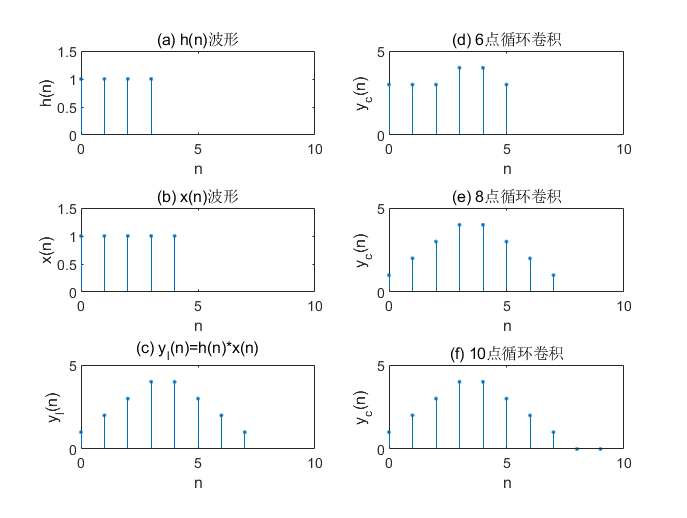四、用DFT计算循环卷积
设$h(n)$和$x(n)$的长度分别为N和M，其L点循环卷积为
$y_c(n)=\Big[\sum_{m=0}^{L-1}h(m)x\big((n-m)\big)_L\Big]R_L(n)$
且
$\begin{cases} H(k)=DFT[h(n)]_L\\ X(k)=DFT[x(n)]_L \end{cases}\qquad 0\leq k \leq L-1，L≥max[N,M]$
则由DFT的时域循环卷积定理有
$Y_c(k)=DFT[y_c(n)]_L=H(k)X(k) \qquad 0\leq k \leq L-1$
由此可见，循环卷积既可以在时域直接计算，也可以按照下面的计算框图在频域计算。
由于DFT有快速算法，当L很大时，在频域计算循环卷积的速度快得多，因而常用DFT（FFT）计算循环卷积。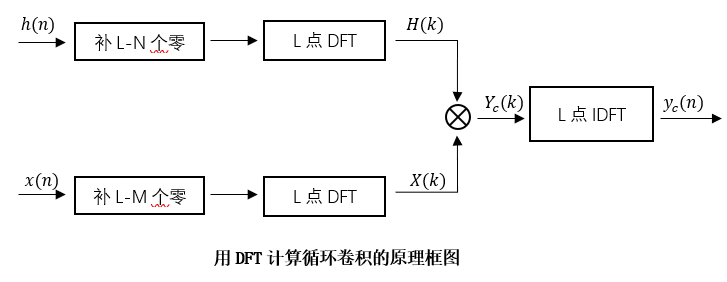DFT只能直接用来计算循环卷积，然而在实际应用中，为了分析时域离散线性时不变系统或者对序列进行滤波处理等，需要计算两个序列的线性卷积。与计算循环卷积一样，为了提高运算速度，也希望用DFT（FFT）计算线性卷积。
五、用DFT计算线性卷积
取L≥N+M-1，则可按照上述框图用DFT（FFT）计算线性卷积。
六、MATLAB实现
1. 利用MATLAB的conv()函数计算线性卷积
% x1的长度N=5，x2的长度M=3,那么x1与x2线性卷积的输出序列长度L=N+M-1=7
% 可以验证，输出结果与我们使用列表法得到的结果一致
x1=[1,1,3,4,2];
x2=[1,3,2];
y=conv(x1,x2);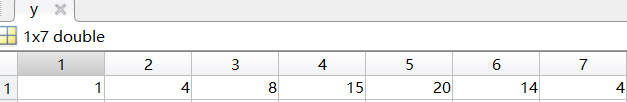2. 利用矩阵相乘原理计算循环卷积
function [y] = circle_convolution(x1,x2,L)
% circle_convolution 函数用于计算两个有限长序列的L点循环卷积
% x1: 长度为N的有限长序列
% x2: 长度为M的有限长序列
% L:  循环卷积的长度
% y:  x1与x2的L点循环卷积结果，L≥max(N,M);

%********** 构造L点循环卷积矩阵 **********%
N=length(x1);
M=length(x2);

x_1=[x1 ,zeros(1,L-N)] ;
x_2=[x2 ,zeros(1,L-M)] ;
x=zeros(L,L);
h=circshift ( fliplr (x_1) ,1);  % 得到x1(n)的循环倒相序列
for i=0:L-1
x(i+1,:)= circshift(h,i);    % x即为L点循环卷积矩阵
end
%********** 矩阵相乘计算循环卷积 **********%
x_2=x_2';     % 将补零后的x2转置为列向量
y=x*x_2;      % 矩阵相乘
end

% 调用circle_convolution函数计算循环卷积
x1=[1,1,3,4,2];
x2=[1,3,2];
L=7;
y=circle_convolution(x1,x2,L);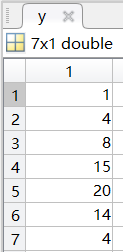当L=9时，结果为：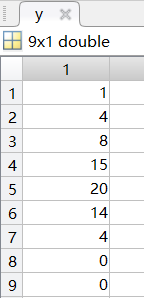当L=5时，结果为：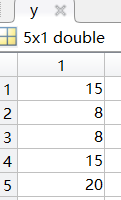这里也进一步验证了线性卷积和循环卷积的关系：
(1) 当L<N+M-1时，循环卷积是线性卷积长度为L的混叠；
(2) 当L=N+M-1时，循环卷积=线性卷积；
(3) 当L>N+M-1时，循环卷积是线性卷积末尾补L-(N+M-1)个零；
3. 调用fft()函数计算循环卷积与线性卷积
% 5点DFT
x1=[1,1,3,4,2];
x2=[1,3,2];

X1=fft(x1,5);
X2=fft(x2,5);
Y=X1.*X2;
y=ifft(Y);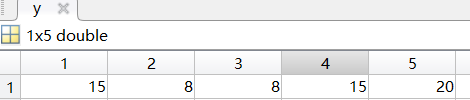% 7点DFT
x1=[1,1,3,4,2];
x2=[1,3,2];

X1=fft(x1,7);
X2=fft(x2,7);
Y=X1.*X2;
y=ifft(Y);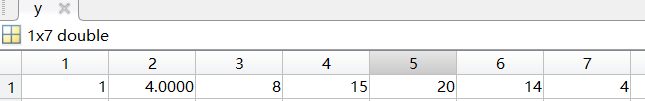% 9点DFT
x1=[1,1,3,4,2];
x2=[1,3,2];

X1=fft(x1,9);
X2=fft(x2,9);
Y=X1.*X2;
y=ifft(Y);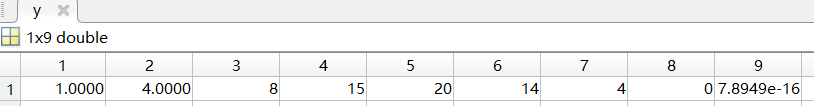`
展开全文• 第二种方法是通过循环卷积和线性卷积之间的关系来运算的。 其原理我就不细讲了，课本上都有，然后用的方法也是我们做计算题时最常用的方法。 话不多说，看程序： function y = circonv(x1,x2,n) %计算循环卷积 ...matlab 数字信号处理
• 掌握循环卷积和线性卷积两者之间的关系。二、实验原理 数字滤波器根据系统的单位脉冲响应h(n) 是有限长还是无限长可分为有限长单位脉冲响应(Finite Impulse Response)系统(简记为FIR系统)和无限长单位脉冲响应...
• 将M点序列x(n)，L点序列h(n)分别作扩展，构造新序列x’(n)，h’(n)，使得长度N满足如下条件根据时域循环卷积定理，x(n)与h(n)的线性卷积可以用循环卷积来代替。即根据式(9)，给出了一种基于快速傅里叶FPGA
• 2、进一步掌握循环卷积和线性卷积两者之间的关系。 二、实验原理 MATLAB中计算序列的离散傅里叶变换和逆变换是采用快速算法，利用fft和ifft函数实现。 1、[x]=fft(x, N) 输入参数：为待计算DFT的序列，N为序列的...
• 所谓神经网络结构就是指不同神经元之间连接结构，一个最简单神经网络结构输出就是所有输入加权。输入权重就是神经元参数，神经网络优化过程就是优化神经元中参数取值过程。 •在深度学习（这个...
• 掌握循环卷积和线性卷积两者之间的关系。 二、实验原理 数字滤波器根据系统的单位脉冲响应h(n) 是有限长还是无限长可分为有限长单位脉冲响应（Finite Impulse Response）系统（简记为FIR系统）和无限长单位脉冲响应...算法 信号处理
• 掌握循环卷积和线性卷积两者之间的关系。二、实验原理 数字滤波器根据系统的单位脉冲响应h(n) 是有限长还是无限长可分为有限长单位脉冲响应(Finite Impulse Response)系统(简记为FIR系统)和无限长单位脉冲响应...
• (2)同样，根据循环卷积和线性卷积的关系以及题目给定的条件，进行27个点循环卷积就能保证 2．设环移位的关系，即是两个长度相同的序列，并且都是偶对称序列，两者之间还是循若以这两个序列分别作为两个线性相位FIR ...
• ## 线性分组码生成矩阵例题

万次阅读 多人点赞 2019-03-05 09:46:57
这块属于信道编码部分，信道编码分为线性分组码（循环码）非分组码（卷积码）。 生成矩阵（n，k）线性分组码生成矩阵为 k 行 n列，如 （7,3），即信息位 3位，码长 7位。监督矩阵生成矩阵正相反，互为转置...通信原理
• 本次主要记录一个简单例子，以后会慢慢深入，包括多层神经网络，卷积神经网络，循环神经网络，自编码网络，深度神经网络对抗神经网络。 实例描述 假设有一组数据集，其中x与y对应关系为：y = 3x + 0.5。我们...神经网络 人工智能 TensorFlow
• 题目一、FFT对连续信号进行谱分析 若信号由20Hz，220 Hz750Hz三个正弦频率成分构成，试用FFT计算其频谱并画出其幅度谱（要求横坐标...（1） 线性卷积与循环卷积的关系； （2） 什么条件下，线性卷积循环卷积相等？信号与系统
• ## LCNN论文阅读笔记

千次阅读 2017-04-08 20:58:10
训练网络就变成了去训练一个字典一些线性组合关系。字典规模就需要找到效率准确率之间平衡。LCNN解决了两个问题：训练集数目少问题减少循环迭代次数问题。他减少迭代次数办法是让字典可以神经网络
• 3.监督学习：给定一组数据，知道正确的输出结果是什么样子，并且知道输入输出之间有一种特定的关系，分为回归分类问题。 无监督学习：不知道结果是什么样子，通过聚类的方式从数据中提取一个特殊的结构。 4....深度学习概论
• 　＋掌握利用循环卷积计算线性卷积的方法；　＋掌握用DFT分析确定信号频谱的方法；　＋理解DFT应用中出现的一些问题原因及解决问题的方法。　5.快速傅立叶变换（讲课6学时，实验2学时）　基本要求　＋掌握基2 FFT...
• 提出一个轻量级的、可端到端训练的全局推理单元GloRe来高效推理image regions之间的关系，增强了模型上下文建模能力 Cityscapes Mean IoU=78.4% PSPNet (res101) - PASCAL Context Mean IoU=48.9 GloRe (res101)...
• 本书特色有二，旨在提高读者问题求解能力，使读者能够理解算法设计过程思想：一是强调算法设计创造性过程，注重算法设计背后创造性思想，而不拘泥于某个具体算法详细讨论；二是将算法设计类比于定理...
• 循环的角度求均值 数字信号处理中各种频率关系 数理统计知识整理——回归分析与方差分析 滤波器设计指标 白话压缩感知 矩阵特征值分解与奇异值分解含义解析及应用 自适应含噪信号过零率算法 基于谱减法声音去噪 读...